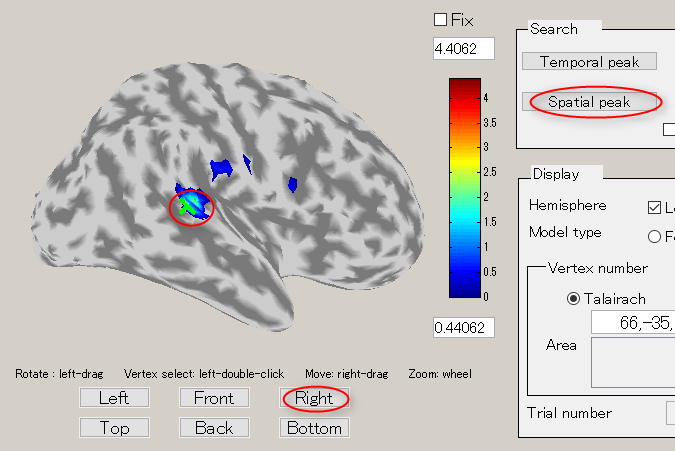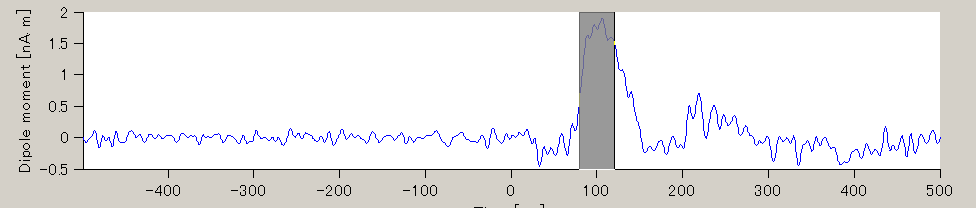# Tutorial

## 1. Introduction

This tutorial aims to get started with VBMEG through the analysis of a sample data set using GUI. The tutorials suppose you have basic knowledge on MEG/EEG and current source imaging.

### 1. Scenario

A subject participated the auditory experiment in which 3.2 kHz pure tone is delivered to a subject left ear. Evoked magnetic fields were measured with Yokogawa MEG system. T1-weighted MRI was measured with Simens Trio 3T, which is used to construct an individual subject brain model.

In this tutorial, we start from importing preprocessed MEG data and T1-MRI data. MEG data was preprocessed using MEGLABORATORY (YOKOGAWA SOFTWARE) to obtain trial-average MEG data. T1-MRI was preprocessed using FreeSurfer to obtain the subject's cortical surface. In addition MEG sensor positions were coregistered to the subject T1-image using our positioning software.

### 2. Directory

• Programs and input data are assumed to be stored as following:
```D:\vbmeg(VBMEG program directory)
D:\data(input data directory)
│  3D.nii
│
├─FS
│  ├─bem
│  │      lh.curv.asc
│  │      lh.inflated.asc
│  │      lh.smoothwm.asc
│  │      lh.sphere.reg.asc
│  │      rh.curv.asc
│  │      rh.inflated.asc
│  │      rh.smoothwm.asc
│  │      rh.sphere.reg.asc
│  │
│  └─label
│          lh.cortex.label
│          rh.cortex.label
│
└─Yokogawa
A008a_BC_0.5HPF_100LPF_trig437_label4.ave
marker1.pos.mat
```

### 3. File

• MEG data file(A008a_BC_0.5HPF_100LPF_trig437_label4.ave)
• Sensor alignment file(marker1.pos.mat)
• Structural MRI image file(3D.nii)
• Cortical model file(lh.(curv/inflated/smoothwm).asc, rh.(curv.inflated/smoothwm).asc)

MEG Data file

• MEG data file(Yokogawa Electric Corporation format)
• Averaged brain activity when a 3.2 kHz pure tone is presented to the left ear

Sensor alignment file

• Information file to align MEG sensor coordinate system with subject’s structural MRI image coordinate system
• Created by positioning program.

Details of MRI structure image file

Details of cortical model file

## 2. Starting project

### 1. Setting a path

• Start MATLAB and load VBMEG as follows: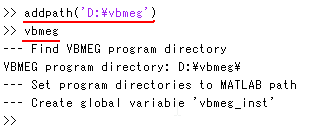### 2. Creating project

1. Start project_mgr from command line.

>> project_mgr

2. Select [File]->[New project].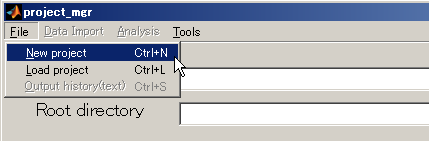3. Input project name and push the select button.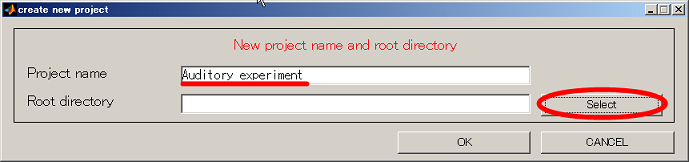4. Change current directory to "D:". When you press the "make dir" button the dialog to input the name of the directory appears. Input "Auditory" and press the OK button.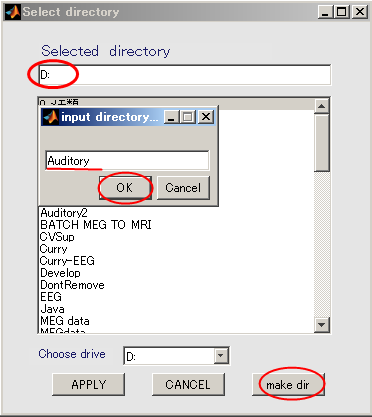5. The working directory(D:\Auditory) in the dialog that appears, and push APPLY button.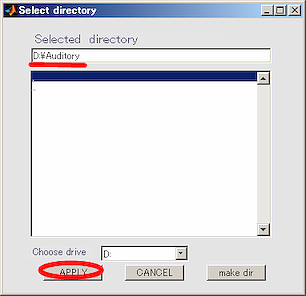6. Press OK button and close the dialog.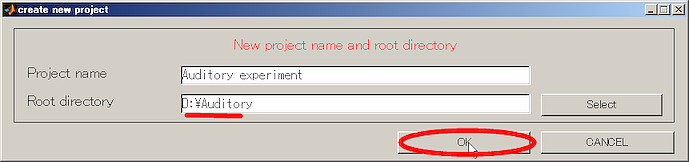7. Select YES in the cofirmation dialog that appears.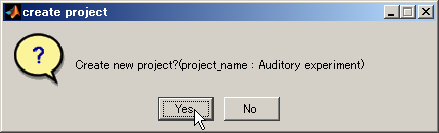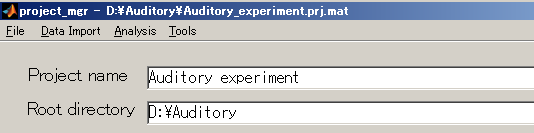## 3. Brain modeling

### 1. Importing a cortical model

1. Select [Data Import]->[Import cortical model].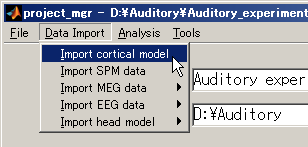2. Press the "Set Freesurfer subject directory" to set coritcal model files.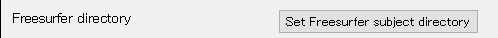In the appearing dialog, choose "D:\data\FS" and press the APPLY button.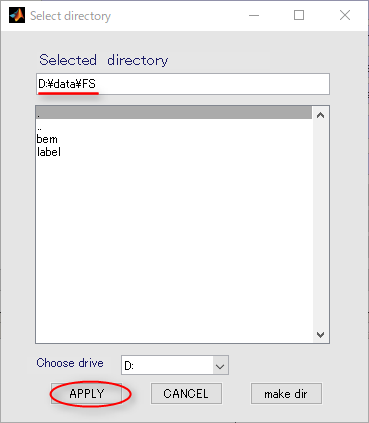Then, freesurfer files are automatically set as below.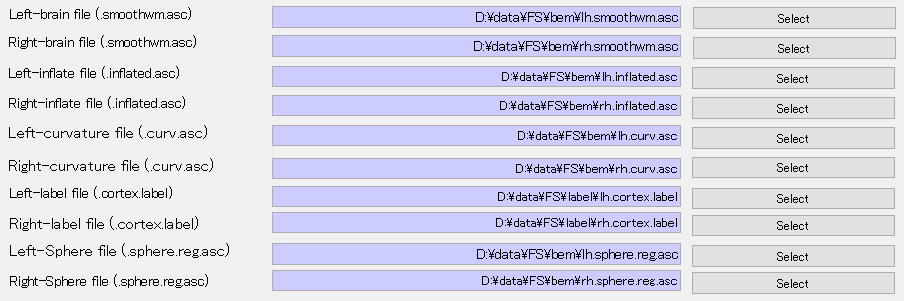3. Press the select button for MRI file.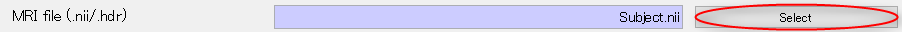In the appearing dialog, choose "D:\data\3D.nii" and press the OK button.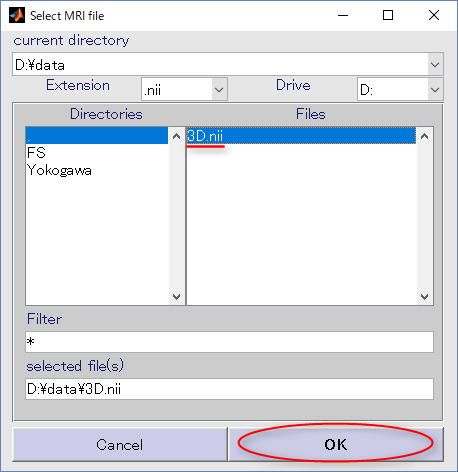Then MRI file is set as below.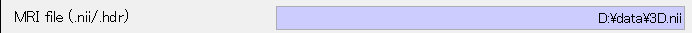4. Specifying output directory (D:\Auditory\brain)
• Press Select button.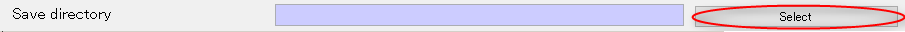• When you press the "make dir" button the dialog to input the name of the directory appears. Input "brain" and press the OK button.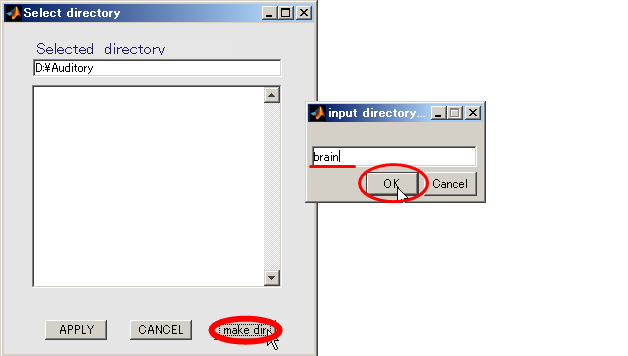• Press the APPLY button.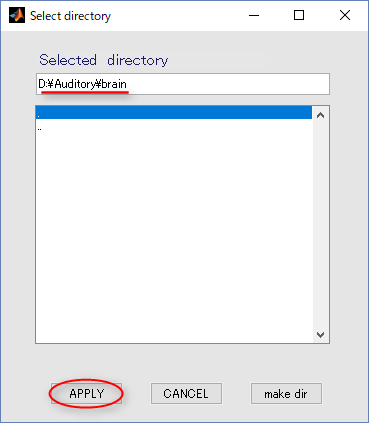• The output file name is updated.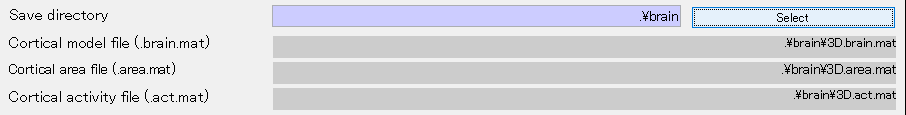5. Press Exec button and wait for approximately 20 minutes. When the importation of cortical model is complete the results will be displayed.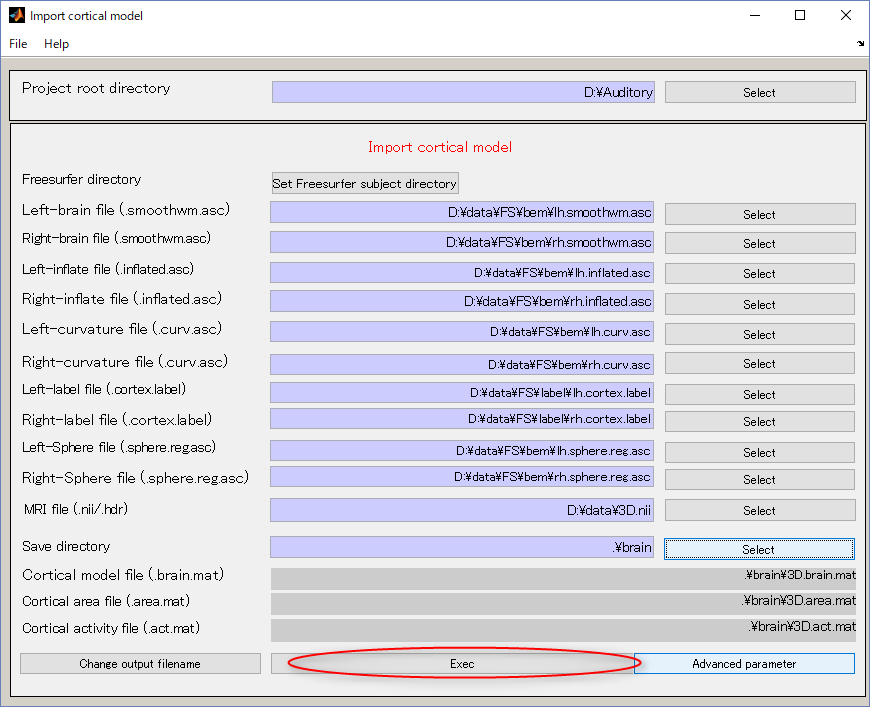The following figure shows the displayed results.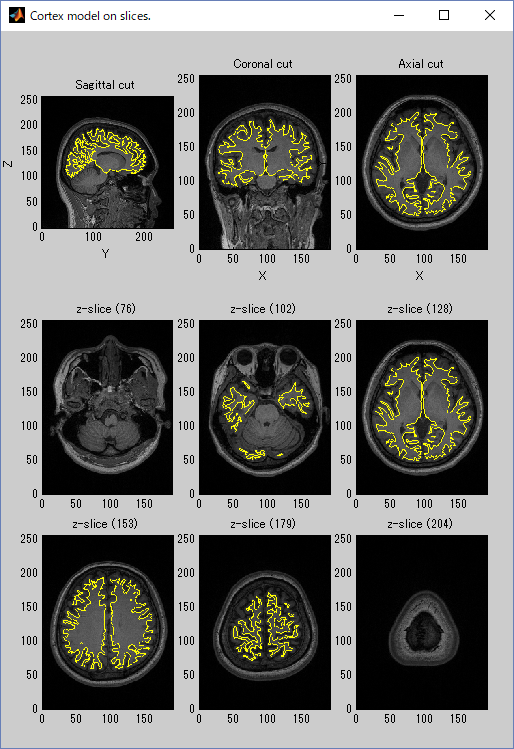## 4. MEG/EEG preprocessing

### 1. Importing MEG data

1. Select [Data Import]->[Import MEG data]->[Yokogawa].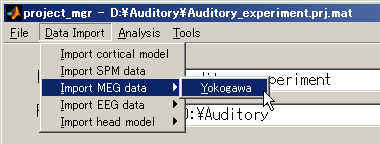2. Specify Yokogawa MEG file.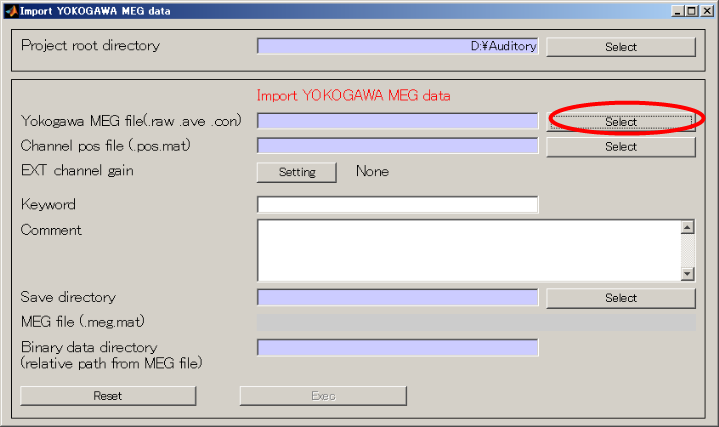3. Change the extension to .ave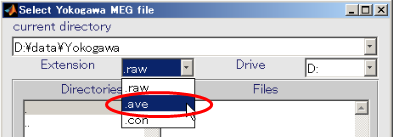4. Select Yokogawa MEG file and press OK.(D:\data\Yokogawa\A008aBC0.5HPF100LPFtrig437_label4.ave)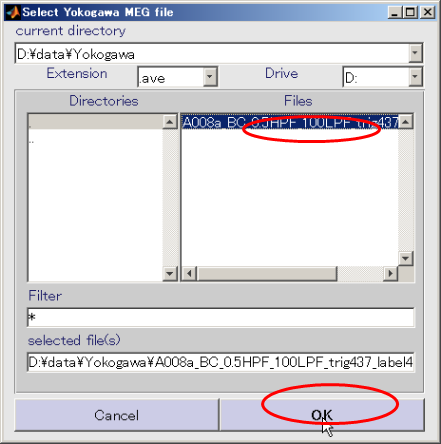5. Press select button and select sensor alignment file(D:\data\Yokogawa\marker1.pos.mat).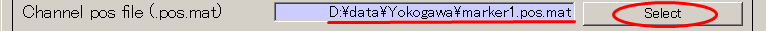6. Create output destination(D:\Auditory\meg).
• Press the Select button.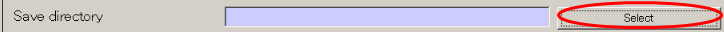• When you press the "make dir" button the dialog to input the name of the directory appears. Input "meg" and press the OK button.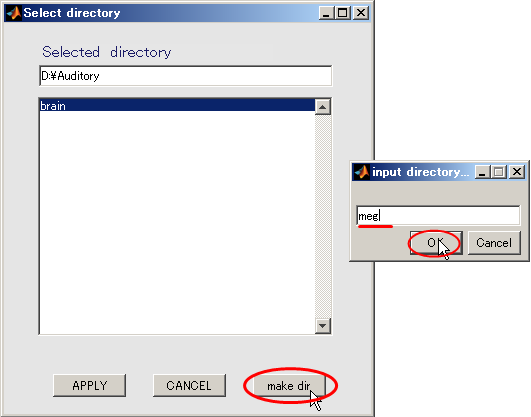• Press the APPLY button.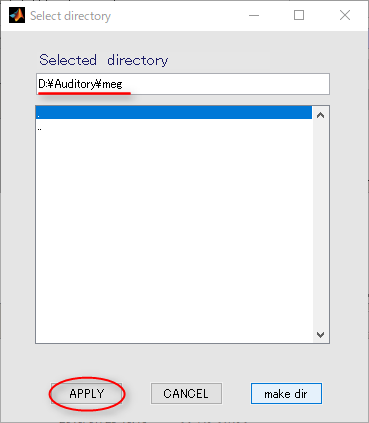• The output file name is updated.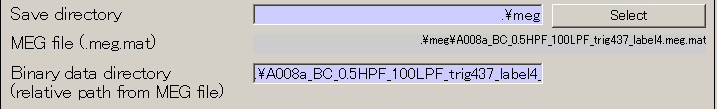7. Press Exec button. The MEG data import will be completed in approximately 10 seconds.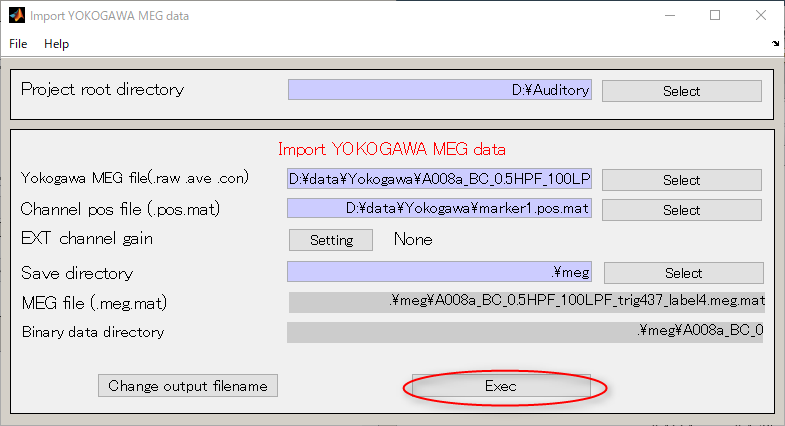<<MATLAB Command window>>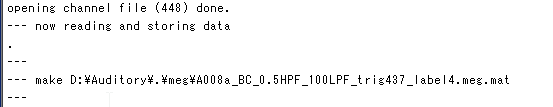### 2. View MEG

meg_file = 'D:\Auditory\meg\A008a_BC_0.5HPF_100LPF_trig437_label4.meg.mat';

job_plot_meg(meg_file);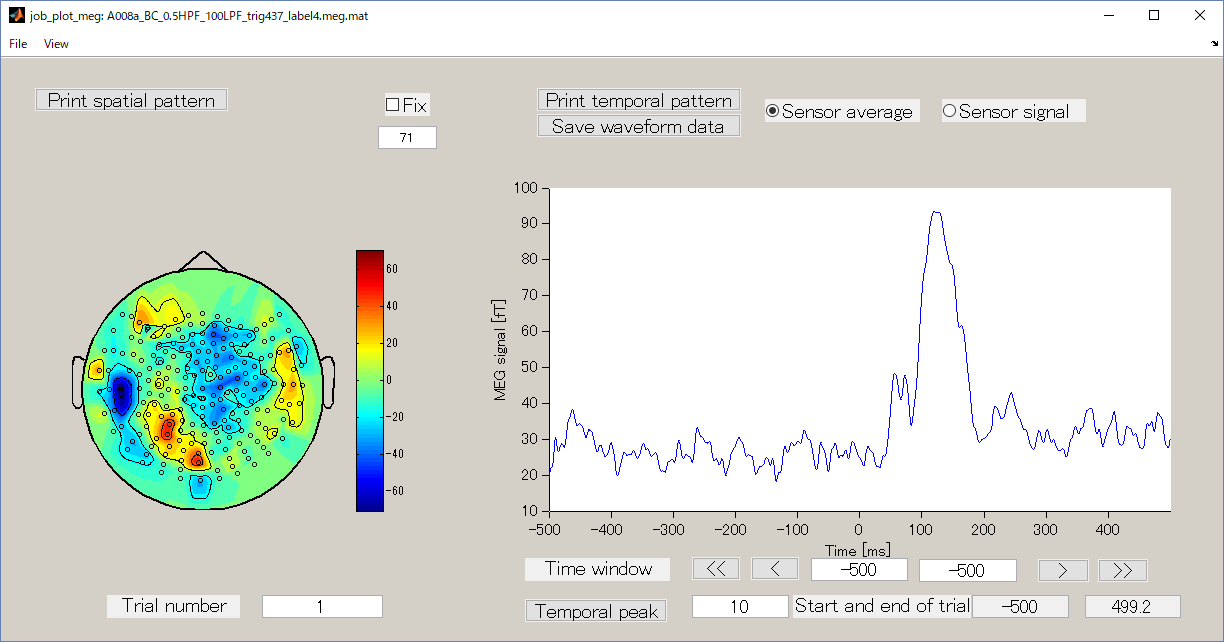## 5. Source imaging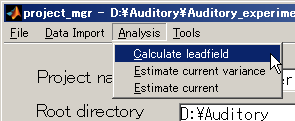2. Specify MEG file(D:\Auditory\meg\A008a_BC_0.5HPF_100LPF_trig437_label4.meg.mat).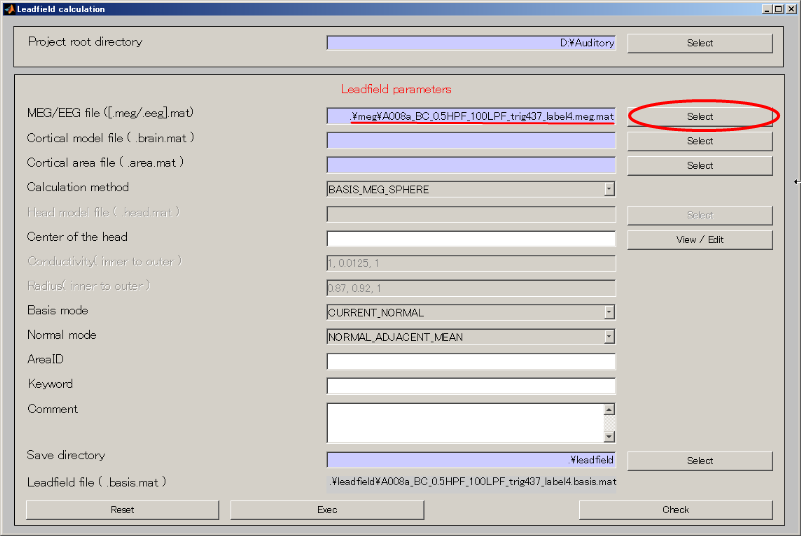3. Specify Cortical model file(D:\Auditory\brain\3D.brain.mat).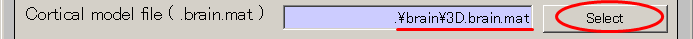• Push Select button.• When you press the "make dir" button the dialog to input the name of the directory appears. Input "leadfield" and press the OK button.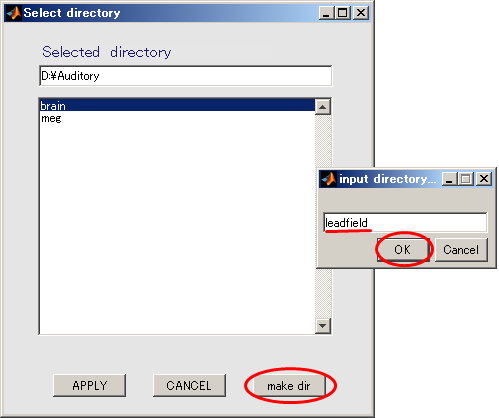• Press APPLY button.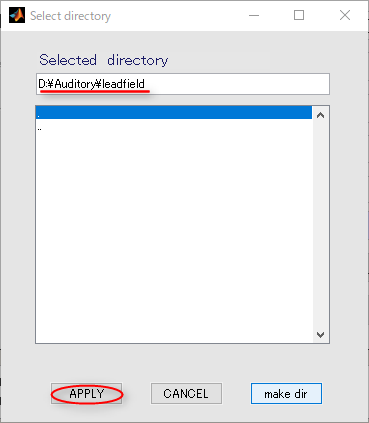• The output file name is updated.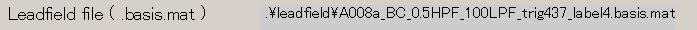5. Press Exec button. The leadfield calculation will be completed in 20 to 30 seconds.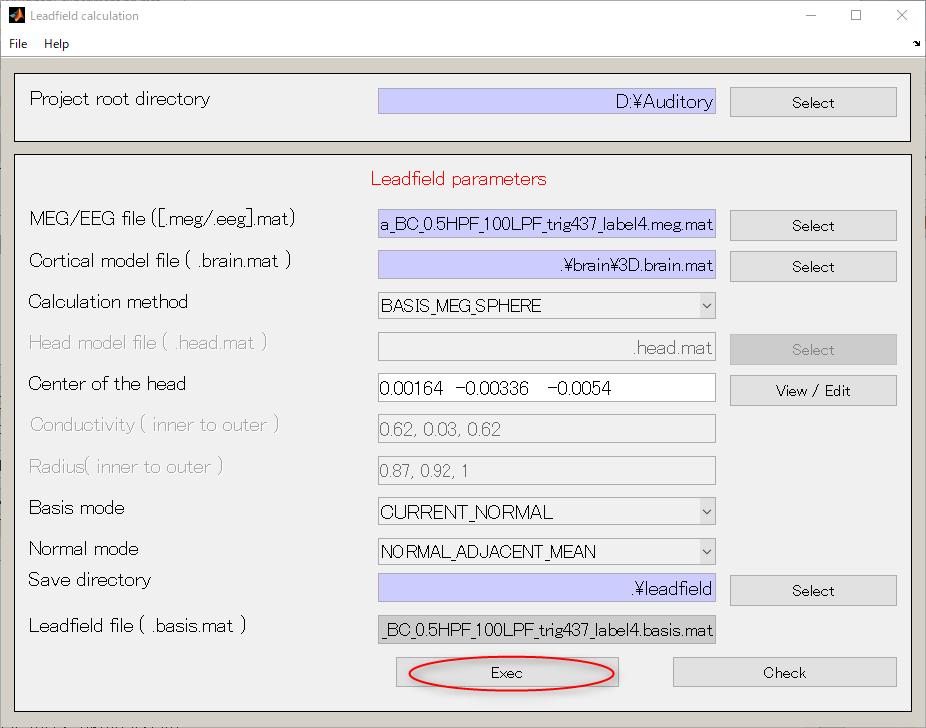<<MATLAB Command window>>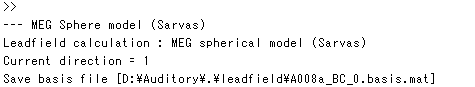### 2. Estimating current variance

1. Select [Analysis]->[Estimate current variance].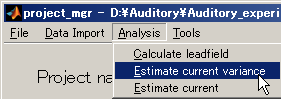2. Specify Cortical model file(D:\Auditory\brain\3D.brain.mat).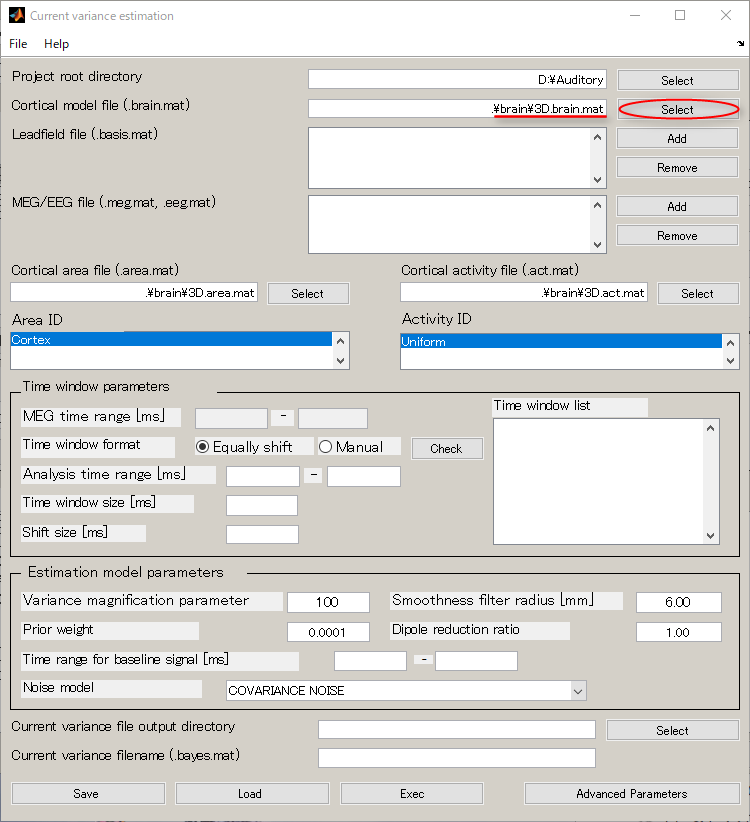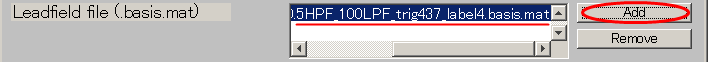4. Specify MEG file(D:\Auditory\meg\A008a_BC_0.5HPF_100LPF_trig437_label4.meg.mat).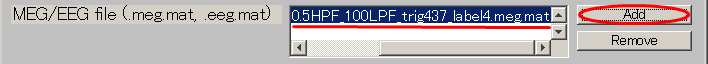5. Specify Cortical area file(D:\Auditory\brain\3D.area.mat).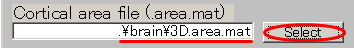6. Specify Cortical activity file(D:\Auditory\brain\3D.act.mat).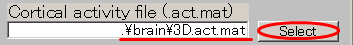7. Set the Variance magnification parameter to 10.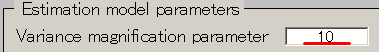8. Set the Prior weight parameter to 0.018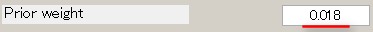9. Set the Dipole reduction ratio to 0.2.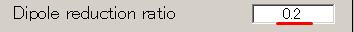10. Create output destination(D:\Auditory\bayes).
• Press Selectbutton.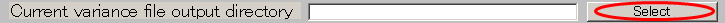• When you press the "make dir" button the dialog to input the name of the directory appears. Input "bayes" and press the OK button.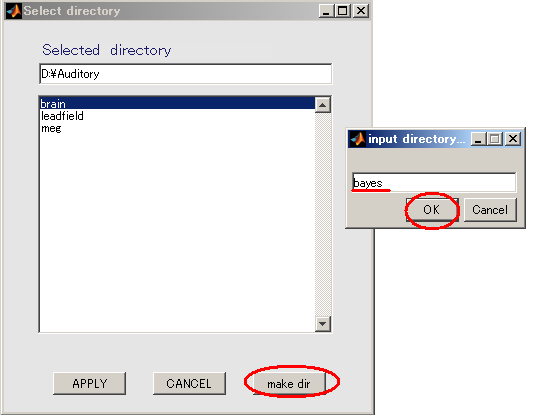• Press the APPLY button.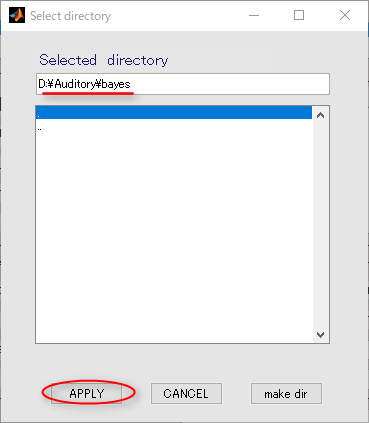11. Input the name of the output file.

auditory_left[ENTER] will make it possible to add the extension:.bayes.mat automatically to the file name.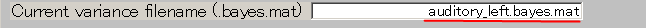12. Press "Exec" button and the estimate will be complete in approximately 20 minutes.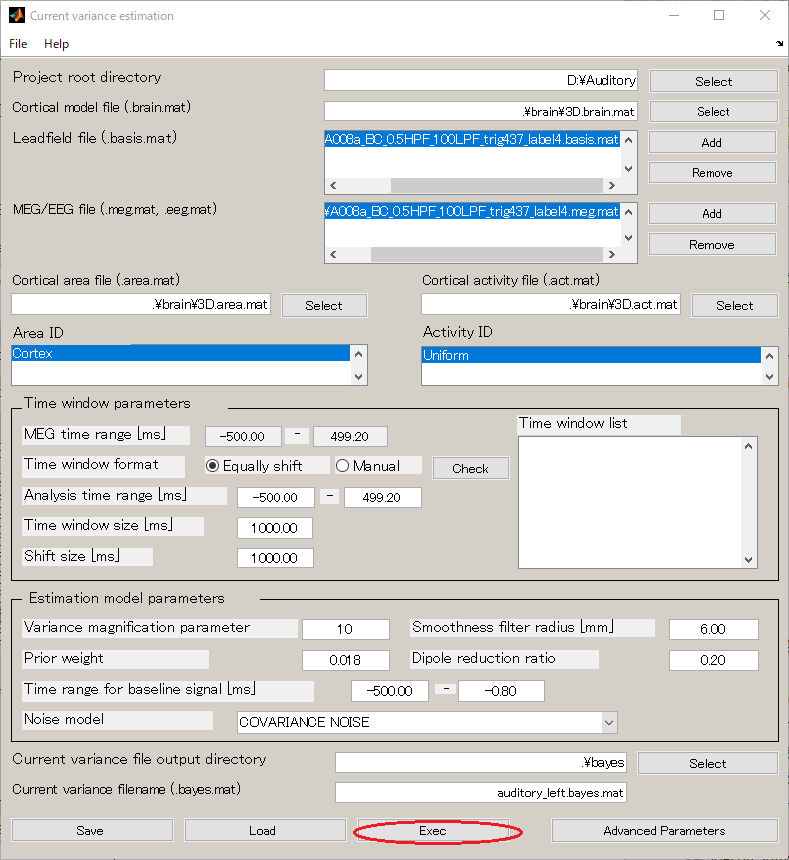<<MATLAB Command Window>>

```    Noise model: full covariance
Load MEG data for noise estimate [D:\Auditory\.\meg\A008a_BC_0.5HPF_100LPF_trig437_label4.meg.mat]
Session 1: -500.0 - -0.8[ms]
Number of basis files (= Number of sessions): 1
Area ID: Cortex
Number of vertices: 9336
--- Reduce cortex
max face ratio=0.200000
edge index number 30000
mesh simplificaton in progress ...

Edges collected: 15000
Edges proccessed: 4011
Edges collapsed: 4000

Edges not collapsed due to topological constrians: 10
Edge not collapsed due to cost computation constrians: 0
Edge not collapsed due to placement computation constrians: 0

Finished...
12000 edges removed.
3000 final edges.
max face ratio=0.200000
edge index number 29994
mesh simplificaton in progress ...

Edges collected: 14997
Edges proccessed: 4015
Edges collapsed: 4000

Edges not collapsed due to topological constrians: 14
Edge not collapsed due to cost computation constrians: 0
Edge not collapsed due to placement computation constrians: 0

Finished...
12000 edges removed.
2997 final edges.
Number of reduced vertices: 1840
--- Spatial smoothing filter calculation
R = 6.00e-03, Rmax= 1.20e-02
Number of vertices = 1840
Number of vertices in expanded area = 9568
Basis file for session 1: D:\Auditory\.\leadfield\A008a_BC_0.5HPF_100LPF_trig437_label4.basis.mat
Number of basis files (= Number of sessions): 1
Area ID: Cortex
Number of vertices: 9336
--- Reduce cortex
max face ratio=0.200000
edge index number 30000
mesh simplificaton in progress ...

Edges collected: 15000
Edges proccessed: 4011
Edges collapsed: 4000

Edges not collapsed due to topological constrians: 10
Edge not collapsed due to cost computation constrians: 0
Edge not collapsed due to placement computation constrians: 0

Finished...
12000 edges removed.
3000 final edges.
max face ratio=0.200000
edge index number 29994
mesh simplificaton in progress ...

Edges collected: 14997
Edges proccessed: 4015
Edges collapsed: 4000

Edges not collapsed due to topological constrians: 14
Edge not collapsed due to cost computation constrians: 0
Edge not collapsed due to placement computation constrians: 0

Finished...
12000 edges removed.
2997 final edges.
Number of reduced vertices: 1840
--- Spatial smoothing filter calculation
R = 6.00e-03, Rmax= 1.20e-02
Number of vertices = 1840
Number of vertices in expanded area = 9568
Basis file for session 1: D:\Auditory\.\leadfield\A008a_BC_0.5HPF_100LPF_trig437_label4.basis.mat
Number of sessions          : 1
Time window                 : -500.0 - 499.2 [ms]
MEG data file (session  1)  : D:\Auditory\.\meg\A008a_BC_0.5HPF_100LPF_trig437_label4.meg.mat
Number of sensors           : 400
Number of trials            : 1
--- Check variable consistency is OK
Noise model: spherical
Load MEG data for noise estimate [D:\Auditory\.\meg\A008a_BC_0.5HPF_100LPF_trig437_label4.meg.mat]
Session 1: -500.0 - -0.8[ms]
Number of sessions          : 1
Time window                 : -500.0 - -0.8 [ms]
MEG data file (session  1)  : D:\Auditory\.\meg\A008a_BC_0.5HPF_100LPF_trig437_label4.meg.mat
Number of sensors           : 400
Number of trials            : 1
--- Initial VB-update iteration = 100
--- Total update iteration      = 100
Sensor noise variance is estimated
Background current variance is estimated
Set prior fMRI activity pattern

--- New VBMEG estimation program ---

--- Initial VB-update iteration = 1000
--- Total update iteration      = 1000
Tn=  1, Iter=  50, FE=16184522.733988, Error=3.111705e-01
Tn=  1, Iter= 100, FE=16184692.961707, Error=3.119022e-01
Tn=  1, Iter= 150, FE=16184715.548904, Error=3.119734e-01
Tn=  1, Iter= 200, FE=16184723.515984, Error=3.119921e-01
Tn=  1, Iter= 250, FE=16184724.259743, Error=3.119959e-01
Tn=  1, Iter= 300, FE=16184724.376362, Error=3.119950e-01
Tn=  1, Iter= 350, FE=16184724.399412, Error=3.119937e-01
Tn=  1, Iter= 400, FE=16184724.405922, Error=3.119926e-01
Tn=  1, Iter= 450, FE=16184724.408611, Error=3.119917e-01
Tn=  1, Iter= 500, FE=16184724.409974, Error=3.119910e-01
Tn=  1, Iter= 550, FE=16184724.410716, Error=3.119905e-01
Tn=  1, Iter= 600, FE=16184724.411129, Error=3.119902e-01
Tn=  1, Iter= 650, FE=16184724.411361, Error=3.119899e-01
Tn=  1, Iter= 700, FE=16184724.411491, Error=3.119897e-01
Tn=  1, Iter= 750, FE=16184724.411564, Error=3.119895e-01
Tn=  1, Iter= 800, FE=16184724.411605, Error=3.119894e-01
Tn=  1, Iter= 850, FE=16184724.411628, Error=3.119893e-01
Tn=  1, Iter= 900, FE=16184724.411642, Error=3.119892e-01
Tn=  1, Iter= 950, FE=16184724.411649, Error=3.119892e-01
Tn=  1, Iter=1000, FE=16184724.411653, Error=3.119891e-01
Alpha is scaled back by bsnorm
----- Save estimation result in D:\Auditory\.\bayes\audirory_left.bayes.mat
```

### 3. Calculating current

1. Select [Analysis]->[Estimate current].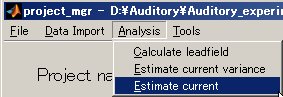2. Specify the current variance file(D:\Auditory\bayes\auditory_left.bayes.mat).

The MEG file is set automatically.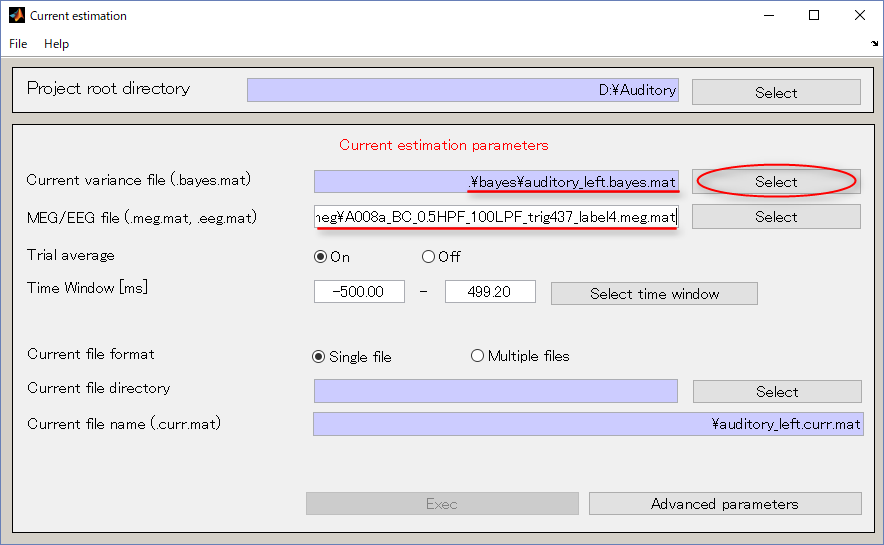3. Specify the output destination(D:\Auditory\current).
• Press Select button.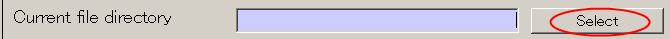• When you press the "make dir" button the dialog to input the name of the directory appears. Input "current" and press the OK button.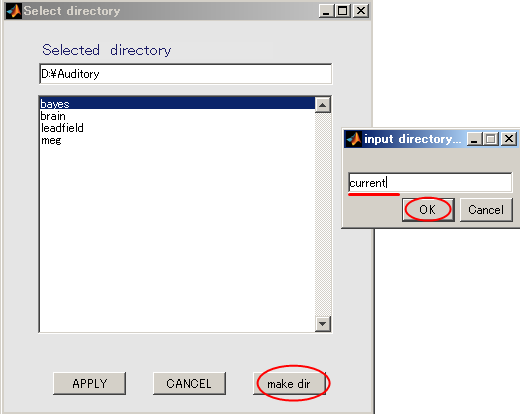• Press the APPLY button.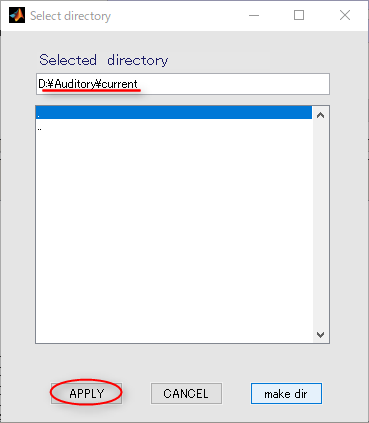• The output file name is updated.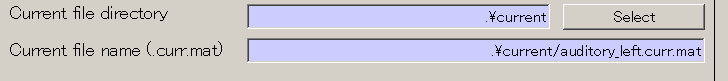4. Press "Exec" button and the current calculation will be complete in 1 minute.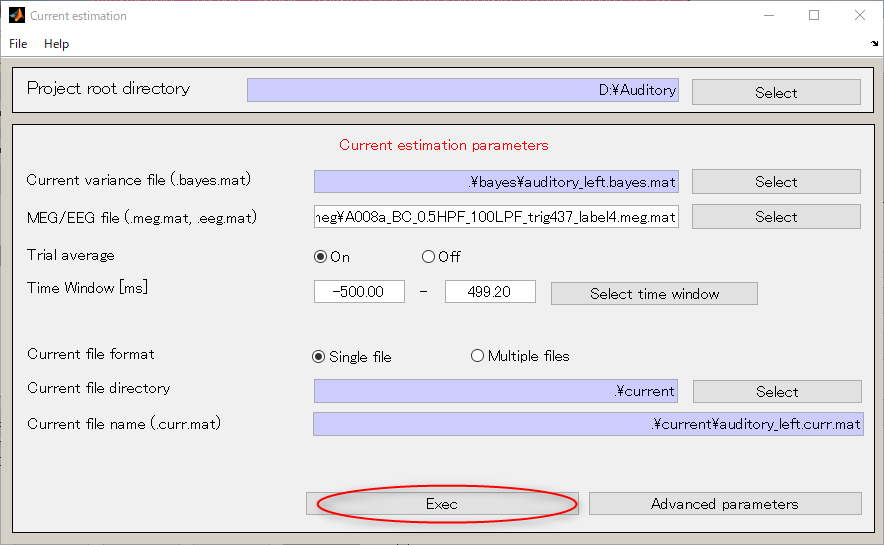<<MATLAB Command Window>>

```    Start current estimation
Number of sessions          : 1
Time window                 : -500.0 - 499.2 [ms]
MEG data file (session  1)  : D:\Auditory\.\meg\A008a_BC_0.5HPF_100LPF_trig437_label4.meg.mat
Number of sensors           : 400
Number of trials            : 1
--- Lead field matrix of focal window
Number of basis files (= Number of sessions): 1
Area ID: Cortex
Number of vertices: 9336
--- Reduce cortex
max face ratio=0.200000
edge index number 30000
mesh simplificaton in progress ...

Edges collected: 15000
Edges proccessed: 4011
Edges collapsed: 4000

Edges not collapsed due to topological constrians: 10
Edge not collapsed due to cost computation constrians: 0
Edge not collapsed due to placement computation constrians: 0

Finished...
12000 edges removed.
3000 final edges.
max face ratio=0.200000
edge index number 29994
mesh simplificaton in progress ...

Edges collected: 14997
Edges proccessed: 4015
Edges collapsed: 4000

Edges not collapsed due to topological constrians: 14
Edge not collapsed due to cost computation constrians: 0
Edge not collapsed due to placement computation constrians: 0

Finished...
12000 edges removed.
2997 final edges.
Number of reduced vertices: 1840
--- Spatial smoothing filter calculation
R = 6.00e-03, Rmax= 1.20e-02
Number of vertices = 1840
Number of vertices in expanded area = 9568
Basis file for session 1: D:\Auditory\.\leadfield\A008a_BC_0.5HPF_100LPF_trig437_label4.basis.mat
Noise model: full covariance
Load MEG data for noise estimate [D:\Auditory\.\meg\A008a_BC_0.5HPF_100LPF_trig437_label4.meg.mat]
Session 1: -500.0 - -0.8[ms]
--- Save estimated current: D:\Auditory\.\current\audirory_left.curr.mat
```

### 4. Viewing current

"View estimated current" from the pop-up menu that appears on the upper right.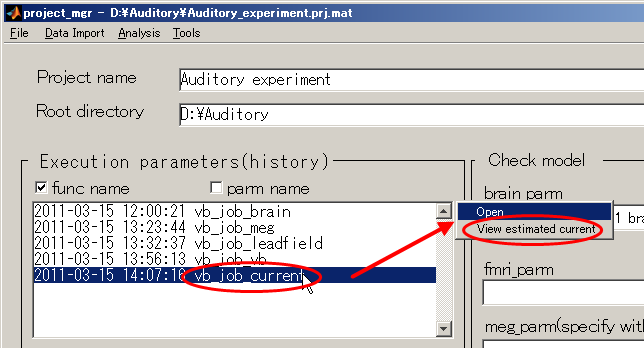Then, dialog appears as below. Press the OK button.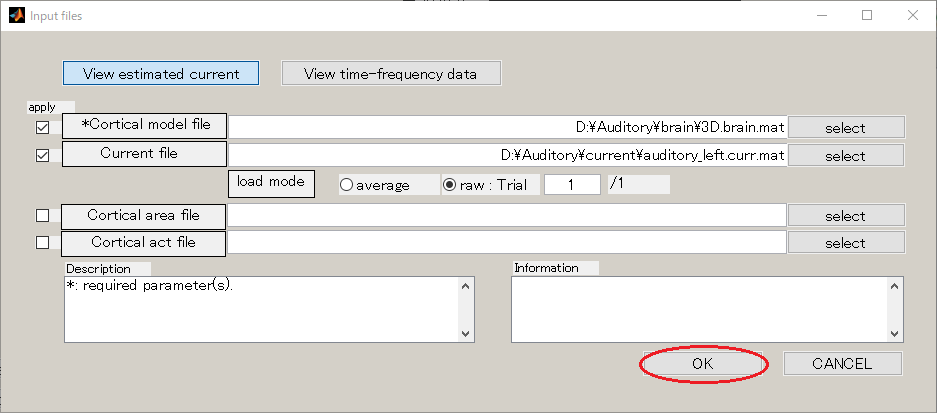2. The GUI to show the estimated current will be displayed. In the spatial pattern display panel on the upper left of the screen, the average current intensity distribution for the selected time of interest (TOI) will be displayed on the inflated brain model. In the current time series display panel at the bottom of the screen, the current intensity time series averaged over the vertex included in the selected region of interest (ROI) will be displayed. The default size of the radius of the ROI is 4 mm.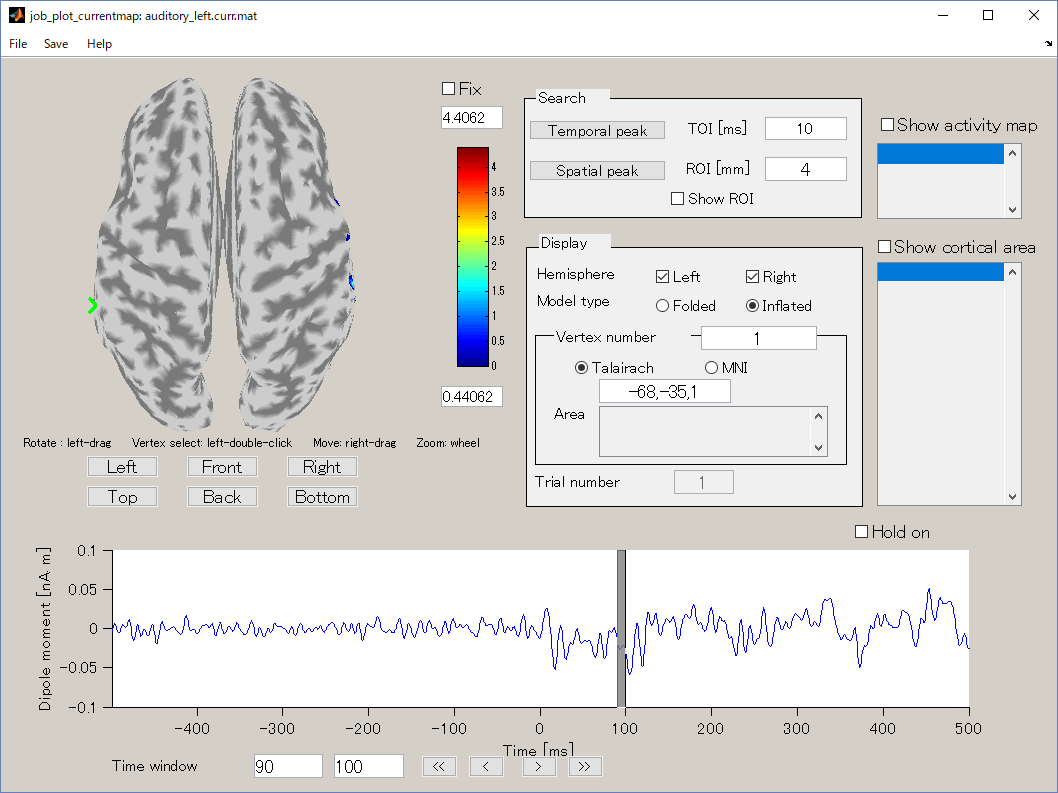3. The TOI can be set by in the "Time window" field. Here, the TOI is set to 80-120 msec which is the latent time of the auditory evoked field (AEF) appearing in the both hemispheres.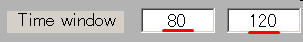The current intensity spatial distribution is updated on the spatial distribution panel along with the setting of the TOI. The TOI appears gray on the time series display panel as shown below: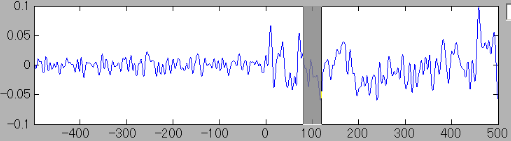4. Press the "Left" button and you can see the cortical model from the left side. Brain activities are shown around the auditory region.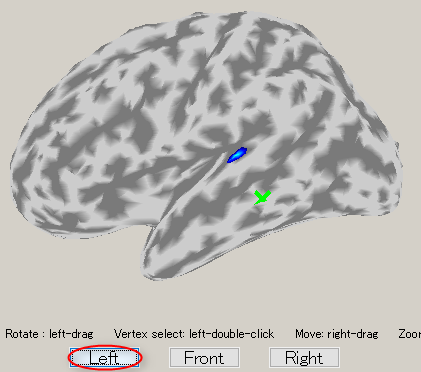5. To choose a vertex (current dipole), left-double-click on a point of the cortical surface model.

Then the green cross moves to the point specified, and the timecourse plot and vertex information is changed to the one on the selected vertex. Here, the brain activity source around the auditory region of the left brain is selected.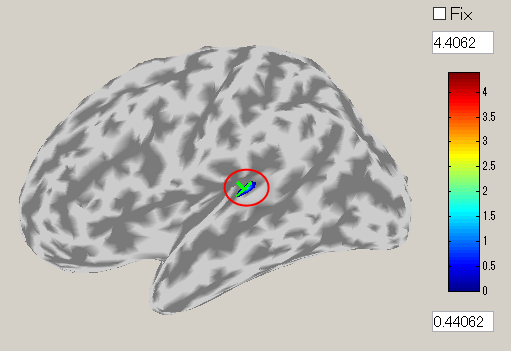The selected vertex index will be shown in "Vertex index" field.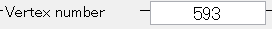The ROI center can also be changed by directly inputing the vertex number to the "vertex index" field.
Current time series of the selected ROI is displayed on the current time series display panel. The y-axis scale varies according to current time series.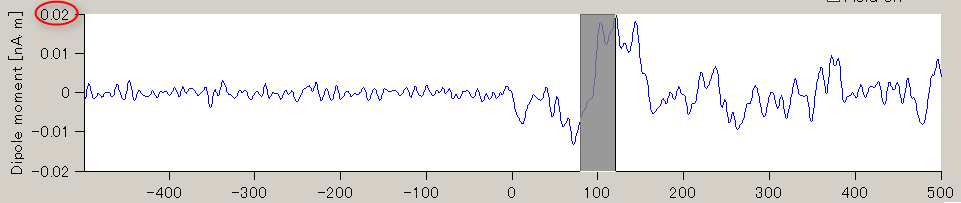6. In order to find the vertex with the highest current intensity, press the "Spatial peak" button. The vertex with the largest time averaged current intensity will be displayed within the ROI and the center of the ROI will be set to this vertex.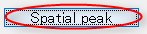Simultaneously, the vertex number in the "Vertex index" field will be updated.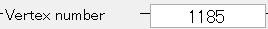The updated ROI current time series will be displayed on the current time series display panel.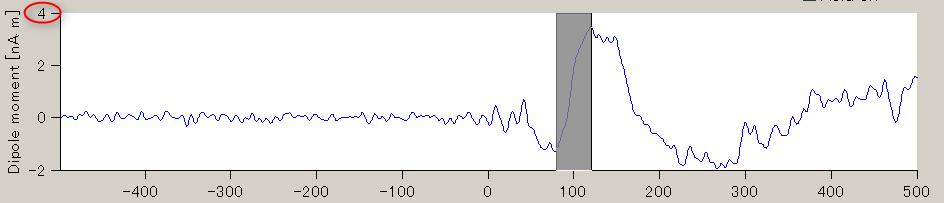7. The estimated current around the auditory region of the right brain can be shown through the same procedure.